# RBSE Solutions For Class 12 Physics Chapter 10: Alternating Current | Textbook Important Questions & Answers

The answers are provided for all the questions of Chapter 10 Physics of RBSE Class 12. Students can go through these questions to understand the concepts better and score well in the board examination and entrance examinations for various professional courses. These solutions are provided by the team of experts to provide the best and accurate solutions to the questions. Do check BYJU’S RBSE Class 12 solutions page to get the answers for textbook questions of all subjects.

## Multiple Choice Questions

Q1: The rms value of alternating current is:

1. Twice of peak value
2. Half of peak value
3. Equal of peak value
4. 1/ time of peak value

Q2: Which of the following is used in ac circuit which shows that the current lead the voltage at the phase

1. Pure resistance
2. Pure inductance
3. Pure capacitor
4. None of these

Q3: The current lags behind the voltage by a phase difference of π/2 radian, when in the circuit is

1. Only resistance
2. Only inductance
3. Only capacitor
4. Both capacitor and resistance

Q4: Capacitor in a circuit:

1. Passes the alternating current
2. Stops the flow of alternating current
3. Passes the direct current
4. Stops the alternating current and passes the direct current

Q5: Power factor of a pure inductor or pure capacitor circuit is

1. One
2. Zero
3. π
4. More than zero

Q1: The AC voltage is given by equation V=200sin100πt. Write its RMS value and frequency.

For AC voltage Vrms = 0.707 x Vm

ω= 2πf

The general expression is given by

Vt=Vmsin(ωt)

Therefore Vrms = 200 x 0.707 =200 V

f = ω/2π = 100π/2π = 50 Hz

Q2: Write down the relation between peak and RMS value of AC current.

Irms = Im

here Irms is the rms value of current

Im is the peak value

Q3: The current in an inductive circuit is given by I=Imsinωt. Give the equation for voltage.

The equation for voltage is given by V= Vmsin(ωt +π/2)t

Q4: The voltage in an AC circuit is given by V =200sin314t. Give the frequency of AC voltage.

ω= 2πf

f = ω/2π =314/(2 x 3.14) = 50 Hz

Q5: How are the inductive and capacitive reactances are affected by increasing AC frequency.

Inductive reactance will increase and capacitive reactance will decrease.

Q6: A coil has inductance of 0.1 H. Find the reactance at 50 Hz.

Given:

Frequency f = 50 Hz

Reactance XL= ωL

XL= 2πfL

= 2 x 3.14x 50 x 0.01

XL = 3.14 Ω

Q7: What will be the phase difference between voltage and current in the series LCR circuit?

The phase difference between voltage and current in the series LCR circuit is between 0 and

Q8: What will be the impedance in series LCR resonant circuit at resonance?

The impedance value is equal to the resistance.

Q9: What will be the phase difference between the voltage across the inductor and voltage across the capacitor in series LCR resonant AC circuit?

The phase difference is equal to 1800

Q10: What will be the value of power factor in AC circuit for inductor, capacitor and resistor?

The power factor in AC circuit for an inductor is zero.

The power factor in AC circuit for the capacitor is zero

The power factor in AC circuit for the resistor is one

Q11: What is the unit of ?

The unit is second

Q12: In series LCR resonant circuit, capacity is changed to 4 times. What will be new inductance for the same resonance frequency?

ω0 = 1/

If the capacitance is increased by 4 times the value of L will be L/4

ω0 =1/

Q13: What will be RMS value of wattless current?

Irms =( Im /)sinӨ

Q14: The ratio of the number of turns in primary and secondary of a transformer is 1:4. What type of transformer is it?

It is a step-up transformer

Q1: Why ac is preferred to dc? Please explain.

The AC is preferred to DC because it can convert high potential to low potential and reduces energy loss.

Q2: 220 V ac is more dangerous than 220 V dc. Why?

220 V ac means the effective or virtual value is 220 V

V0=Vrms

V0 = 1.414 x 220 = 311 volts

The 220 V dc will have the peak value as 220 V itself.

Moreover, the shock caused by AC is attractive and DC is repulsive.

Hence 220 V ac is more dangerous than 220 V dc

Q3: Draw graph between frequency and XL and XC?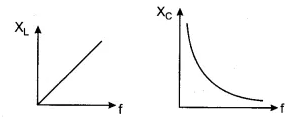Q4: A capacitor stops DC current, while it allows AC current. Why?

DC is blocked because DC has zero frequency, so reactance is infinity. While AC has some frequency, due to which capacitor lets it flow.

Q5: A coil has an ohmic resistance of 6Ω, if its impedance is 10Ω, find inductive reactance XL.

Given

Resistance , R = 6Ω

Impedance, L = 10Ω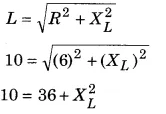XL = = 8

Q6: If fr is the resonant frequency of ac circuit. Give the phase relationship between voltage and current for

(i) f – fr

(ii) f < fr

(iii) f ＞ fr

(i) f – fr when XL = Xc

The circuit is resistive therefore, the phase of current and voltage will be the same.

(ii) f < fr

XL = ωL=2πfL

And XC = 1/ωC = 1/2πfC

When f is less then XL will be less and XC will be large. The circuit is capacitance dominated. Therefore, the current in the circuit leads the voltage by a phase of Φ

(iii) f ＞ fr

When f is large XL will be large and XC will be small. The circuit will be inductance dominated. Therefore, the current in the circuit lags the voltage by a phase of Φ.

Q7: What is bandwidth? Write its expression for LCR series circuit.

Bandwidth is the range of frequencies for which the total power will be more than half of maximum power. It is represented as Δf

Q8: What are half-power frequencies? What will be the current at these frequencies?

Answer: These are the frequencies at which the current in a series RLC circuit is equal of the maximum current. It is also known as cutoff frequencies.

Q9: If the value of resistance and reactance are the same for a coil, what will be its power factor?

The power factor is given by

CosΦ = R/Z

Resistance (R) = Reactance (Z)

Therefore CosΦ = 1

Q10: Write down the expression for impedance, frequency and power factor for a series LCR ac circuit?

For an LCR circuit

Impedance = Resistance

Frequency does not change with resistance

Power Factor CosΦ = 1

Q11: Write down the principle of the transformer and its uses.

Transformer works on the principle of mutual inductance. The transformer is used to convert the high voltage to low voltage or vice versa.

Q12: Find the average value of ac current in its first half positive half cycle.

The average value of ac for the first half cycle:

It is defined as the value of dc which sends the same amount of charge through the circuit in the time equal to that sent by ac in half the time period.

It is given as I=Imsinωt.

Let us assume the current remains constant for the time dt. Then the charge flowing through the circuit in time dt is

dq = Idt

dq = Imsinωtdt

The above equation in integrated to find the charge flowing in time T/2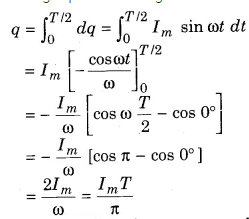The value of ωT = 2π

The average value of ac over one half cycle is

Iav = Charge/Time

= q/(T/2)

= 2q/T

Iav = 2ImT/Tπ

Iav = (2/π)Im = 0.6371Im

Q13: On what factors, the power losses in a transformer depend? How can it be reduced?

1. Magnetic Flux Leakage: There are gaps in the core which reduces the magnetic flux linked with the secondary coil as there is a loss of energy. It can be reduced by winding the primary and secondary coils together
2. Iron loss: This is called the eddy current loss since it is caused by the eddy currents. This can be reduced by using laminated sheets of soft iron.
3. Copper loss: This is the heat energy loss across the copper wire due to resistance. This can be used by using thick copper wire.
4. Hysteresis loss: This loss is caused by repeated magnetisation and demagnetisation of the core. It can be reduced by selecting a material having a narrow hysteresis loop.
5. Magnetostriction: This loss is due to the humming sound produced in the transformer.

Q14: Find the expression for impedance and phase difference between voltage and current in series R-L ac circuit.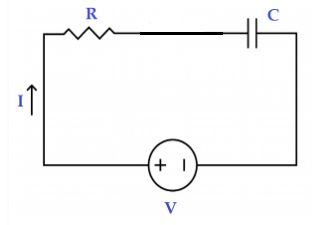An AC voltage V =Vmsinωt is applied to the series combination of resistance and capacitance. If the voltage across C and R is given by VC and VR . The current across the circuit is I. The resultant voltage developed across the RC circuit is VRC. Applying Kirchoff’s law to the circuit we get V- VRC= 0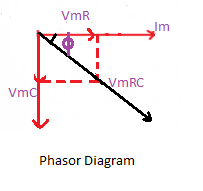From the phasor diagram, we can understand that VmR is in phase with Im. But Vmc lags behind the current by π/2 . The resultant potential across the combination is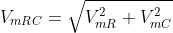——————————–(1)

We know

VmR= Im

VmC=ImXc

Substituting these values equation 1 becomes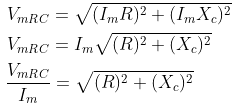Here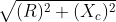is the effective obstacle in the circuit which is called Impedance Z of the circuit.

Therefore Z =Z =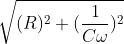From the phasor diagram, it is clear that applying sinusoidal wave to the RC series combination will produce sinusoidal current. But the current leads the voltage by angle Φ.

I = Im Sin(ωt + Φ)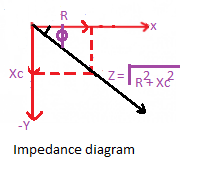In the figure above resistance, R is in the x-axis and Xc is in the y-axis. The resultant is the value of Z, which makes an angle Φ with x-axis.

tan Φ = VmC/VmR = ImXc/ImR = Xc/R

Φ = tan-1 (Xc/R)

## Essay Type Questions

Q1: What do mean by the resonant circuit? Give the required condition for the series LCR resonant circuit and derive the expression for its resonance frequency. Where is this circuit used?

Resonant Circuit: An electric circuit is called a resonant circuit if it has a very low impedance at a certain frequency.

Condition for series LCR resonant circuit

The resonance condition in the circuit arises when the inductive reactance is equal to the capacitive reactance but they cancel each other because they have a phase difference of 1800. The current in the circuit will be maximum.

Expression for its resonance frequency.

Consider an LCR circuit connected in series. The circuit is connected to an AC source.

The impedance of the circuit is given as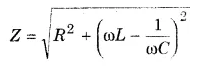If the ω value is varied at a specific frequency ω0

With the condition of resonance XL= XC

Or ω0L = 1/ω0C

ω0 =1/

Angular frequency ω0 = 1/2πf0

f0 = 1/2πω0

Substituting the value of ω0 in above equation we get

f0= (1/2π)

f0 is the resonant frequency

Q2: Derive an expression for power in AC circuit. Also, define the power factor.

Answer: The rate at which energy is absorbed is called the power of the circuit. It is the product of voltage and current in the circuit. The voltage and current will have a phase difference depending on its elements. Therefore, power will also have a phase difference.

The instantaneous current and voltage is given by

V=Vm sinωt

I = Im sin(ωt – Φ)

The current lags the voltage by a potential of Φ

The instantaneous power is given by

P = VI=(Vm sinωt)(Im sin(ωt – Φ))

P = VmImsinωtsin(ωt – Φ)

Simplifying the above equation we get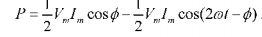The instantaneous power has two components. The average of the cosine function over one complete cycle is zero.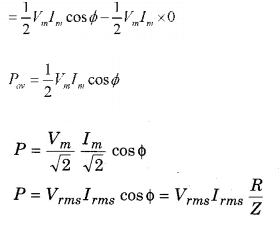The average power depends on voltage, current and cosine of the phase difference between them

The power factor cos Φ = R/Z

The ratio of resistance and impedance in LCR circuit is called power factor